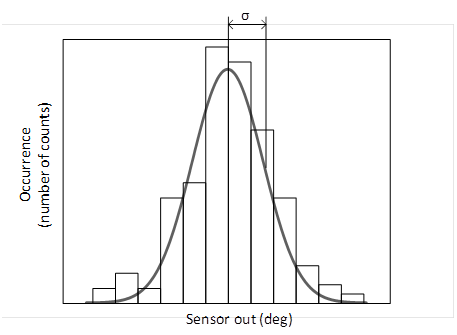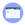## Resolution

Or effective resolution. The smallest quantity distinguishable by a sensor. For a digital output, if the noise is less than 1 LSB, then the resolution is the bit length of the sensor output. If the noise is greater than 1 LSB, the resolution is measured by computing 3 x σ (the standard deviation in degrees) over a large set of data points (e.g. 1000), taken without changing the measurement conditions:

$$resol=3σ$$

Note: for the second case, when the resolution is limited by the noise, the resolution is a measure of the random spread of the sensor output. This is sometimes also called precision. Assuming white noise, the distribution of sensor output when the conditions remain unchanged is a Gaussian function, characterized by a mean value and width σ, equal to the standard deviation.The resolution is often expressed in bit. In this case, ±3 σ corresponds to 1 LSB. Therefore the resolution in bit is  of the full range, divided by 1 LSB:

$$resol(bit)=log_2⁡ {360 \over 6σ}$$